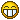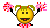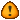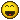Welcome to WSL!

New to the forum? Please read this and this.

## Advanced Pivot macro

JUNE
Fusionista
Posts: 313
Joined: Wed Aug 06, 2014 5:45 am
Been thanked: 4 times
Contact:

### Advanced Pivot macro

AP.jpgMotion Tool
CGS_AdvancedPivot.setting
You do not have the required permissions to view the files attached to this post.

Tags:

SecondMan
Site Admin
Posts: 4828
Joined: Thu Jul 31, 2014 5:31 pm
Answers: 31
Location: Vancouver, Canada
Been thanked: 39 times
Contact:

### Re: Advanced Pivot macro

Oooh that looks pretty awesome!

Looking forward to giving that a whirl - thanks for sharing as always, @JUNE!

PS. added a couple of tags to your topic for easy future retrievalJUNE
Fusionista
Posts: 313
Joined: Wed Aug 06, 2014 5:45 am
Been thanked: 4 times
Contact:

### Re: Advanced Pivot macro

@SecondMan Thanks

SirEdric
Fusionator
Posts: 2377
Joined: Tue Aug 05, 2014 10:04 am
Answers: 12
Real name: Eric Westphal
Been thanked: 4 times
Contact:

### Re: Advanced Pivot macro

Very cool!

Just being creative here...instead of cropping the image one could use the DataWindow from the incoming image directly.
This is Q&D, the expressions in the User tab could certainly be simplified, but ... hey ... it's just a POC after all...Code: Select all

``````{
Tools = ordered() {
Text1 = TextPlus {
CtrlWZoom = false,
Inputs = {
Width = Input { Value = 1920, },
Height = Input { Value = 1080, },
["Gamut.SLogVersion"] = Input { Value = FuID { "SLog2" }, },
Center = Input { Value = { 0.316326530612245, 0.605545724871171 }, },
Font = Input { Value = "Open Sans", },
StyledText = Input { Value = "HELLO", },
Style = Input { Value = "Bold", },
ManualFontKerningPlacement = Input {
Value = StyledText {
Array = {
},
Value = ""
},
},
},
ViewInfo = OperatorInfo { Pos = { 0, 412.5 } },
},
Transform2_4 = Transform {
Inputs = {
Pivot = Input { Expression = "self['p'..self.AdvancedPivot]", },
Angle = Input { Value = 20.22, },
Input = Input {
SourceOp = "Text1",
Source = "Output",
},
AdvancedPivot = Input { Value = 0, },
refWidth = Input { Expression = "1/self.Input.OriginalWidth", },
refHeight = Input { Expression = "1/self.Input.OriginalHeight", },
dw1x = Input { Expression = "self.Input.DataWindow", },
dw1y = Input { Expression = "self.Input.DataWindow", },
dw2x = Input { Expression = "self.Input.DataWindow", },
dw2y = Input { Expression = "self.Input.DataWindow", },
p0 = Input { Expression = "Point(refWidth*dw1x, refHeight*dw2y)", },
p1 = Input { Expression = "Point(refWidth*(dw1x+(dw2x-dw1x)/2), refHeight*dw2y)", },
p2 = Input { Expression = "Point(refWidth*dw2x, refHeight*dw2y)", },
p6 = Input { Expression = "Point(refWidth*dw2x, refHeight*(dw1y+(dw2y-dw1y)/2))", },
p4 = Input { Expression = "Point(refWidth*dw1x, refHeight*(dw1y+(dw2y-dw1y)/2))", },
p10 = Input { Expression = "Point(refWidth*dw2x, refHeight*dw1y)", },
p8 = Input { Expression = "Point(refWidth*dw1x, refHeight*dw1y)", },
p9 = Input { Expression = "Point(refWidth*(dw1x+(dw2x-dw1x)/2), refHeight*dw1y)", },
p5 = Input { Expression = "Point(refWidth*(dw1x+(dw2x-dw1x)/2), refHeight*(dw1y+(dw2y-dw1y)/2))", },
},
ViewInfo = OperatorInfo { Pos = { 440, 412.5 } },
UserControls = ordered() {
AdvancedPivot = {
{ MBTNC_ButtonHeight = 60, MBTNC_AddButton = "┏", MBTNCD_ButtonWidth = 0.2 },
{ MBTNCD_ButtonWidth = 0.2, MBTNC_AddButton = "┳" },
{ MBTNCD_ButtonWidth = 0.2, MBTNC_AddButton = "┓" },
{ MBTNCD_ButtonWidth = 0.4, MBTNC_AddButton = "" },
{ MBTNC_ButtonHeight = 60, MBTNC_AddButton = "┣", MBTNCD_ButtonWidth = 0.2 },
{ MBTNCD_ButtonWidth = 0.2, MBTNC_AddButton = "╋" },
{ MBTNCD_ButtonWidth = 0.2, MBTNC_AddButton = "┫" },
{ MBTNCD_ButtonWidth = 0.4, MBTNC_AddButton = "" },
{ MBTNC_ButtonHeight = 60, MBTNC_AddButton = "┗", MBTNCD_ButtonWidth = 0.2 },
{ MBTNCD_ButtonWidth = 0.2, MBTNC_AddButton = "┻" },
{ MBTNCD_ButtonWidth = 0.2, MBTNC_AddButton = "┛" },
{ MBTNCD_ButtonWidth = 0.4, MBTNC_AddButton = "" },
INP_MaxAllowed = 12,
INP_Integer = true,
INPID_InputControl = "MultiButtonControl",
MBTNC_ShowBasicButton = false,
INP_MaxScale = 12,
INP_Default = 5,
ICS_ControlPage = "Controls",
INP_MinScale = 0,
INP_MinAllowed = 0,
LINKID_DataType = "Number",
MBTNC_ShowName = true,
MBTNC_StretchToFit = false,
MBTNC_ShowToolTip = true,
LINKS_Name = "Advanced Pivot"
},
refWidth = {
INPID_InputControl = "SliderControl",
INP_Integer = false,
LINKID_DataType = "Number",
LINKS_Name = "refWidth",
},
refHeight = {
INPID_InputControl = "SliderControl",
INP_Integer = false,
LINKID_DataType = "Number",
LINKS_Name = "refHeight",
},
dw1x = {
INPID_InputControl = "SliderControl",
INP_Integer = false,
LINKID_DataType = "Number",
LINKS_Name = "dw1x",
},
dw1y = {
INPID_InputControl = "SliderControl",
INP_Integer = false,
LINKID_DataType = "Number",
LINKS_Name = "dw1y",
},
dw2x = {
INPID_InputControl = "SliderControl",
INP_Integer = false,
LINKID_DataType = "Number",
LINKS_Name = "dw2x",
},
dw2y = {
INPID_InputControl = "SliderControl",
INP_Integer = false,
LINKID_DataType = "Number",
LINKS_Name = "dw2y",
},
p0 = {
INPID_InputControl = "OffsetControl",
LINKID_DataType = "Point",
LINKS_Name = "p0",
},
p1 = {
INPID_InputControl = "OffsetControl",
LINKID_DataType = "Point",
LINKS_Name = "p1",
},
p2 = {
INPID_InputControl = "OffsetControl",
LINKID_DataType = "Point",
LINKS_Name = "p2",
},
p6 = {
INPID_InputControl = "OffsetControl",
LINKID_DataType = "Point",
LINKS_Name = "p6",
},
p4 = {
INPID_InputControl = "OffsetControl",
LINKID_DataType = "Point",
LINKS_Name = "p4",
},
p10 = {
INPID_InputControl = "OffsetControl",
LINKID_DataType = "Point",
LINKS_Name = "p10",
},
p8 = {
INPID_InputControl = "OffsetControl",
LINKID_DataType = "Point",
LINKS_Name = "p8",
},
p9 = {
INPID_InputControl = "OffsetControl",
LINKID_DataType = "Point",
LINKS_Name = "p9",
},
p5 = {
LINKS_Name = "p5",
LINKID_DataType = "Point",
INPID_InputControl = "OffsetControl",
}
}
}
}
}``````

JUNE
Fusionista
Posts: 313
Joined: Wed Aug 06, 2014 5:45 am
Been thanked: 4 times
Contact:

### Re: Advanced Pivot macro

@SirEdric
so so cool!
Thank you very much. I learned it.

JUNE
Fusionista
Posts: 313
Joined: Wed Aug 06, 2014 5:45 am
Been thanked: 4 times
Contact:

### Re: Advanced Pivot macro

@SirEdric

self['p'..self.AdvancedPivot]
Is there a detailed description of this expression? THX

SirEdric
Fusionator
Posts: 2377
Joined: Tue Aug 05, 2014 10:04 am
Answers: 12
Real name: Eric Westphal
Been thanked: 4 times
Contact:

### Re: Advanced Pivot macro

JUNE wrote: Tue Sep 10, 2019 8:01 amself['p'..self.AdvancedPivot]
It's just one of the Lua ways to address indices in a table.
So there are those point values called p0, p1, p2, ... in the user tab, which I want to read, depending on the value of the advancedPivot Multibutton Control.
I named them that way, so the number matches the Index returned by advancedPivot.

Normally, to read any of those point you would write `self[p0]` e.g. or (more common) `self.p0`
The rest is just string concatenation of 'p' (because the name of a variable must not start with a number, but a letter) plus the value returned by advancedPivot .
In other words, you could also write write `self['p' .. '0']` to achieve the same as above.
Or `self['p'..self.AdvancedPivot]` to link it to the advancedPivot Multibutton Control....JUNE
Fusionista
Posts: 313
Joined: Wed Aug 06, 2014 5:45 am
Been thanked: 4 times
Contact:

### Re: Advanced Pivot macro

@SirEdric Perfect explanation, I learned new knowledge.